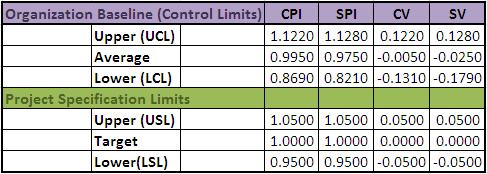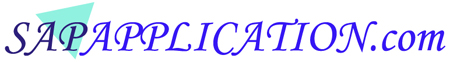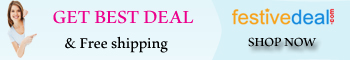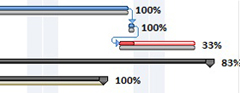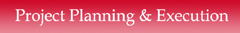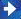Continue.. EVM - CPI, SPI, Cost Variance and Schedule Variance   5. Cost Performance Index (CPI) - Cost Performance Index shows you the utilization       of your resources in the project. It reflects the ratio of actual work done against the       actual cost paid for it. The CPI is calculated as - CPI = Earned Value (EV) / Actual Cost (AC)              CPI < 1 - Over Budget.              CPI > 1 - Under Budget.   6. Cost Variance (CV) - Cost Variance tells you how much over or under budget your       project is running. It is the difference between the Earned Value (EV) and the Actual       Cost (AC) you spent for the work performed. The Cost Variance (CV) is calculated as - CV = Earned Value (EV) - Actual Cost (AC)              CV < 0 (Negative Value) - Over Budget.              CV > 0 (Positive Value) - Under Budget.   7. Schedule Performance Index (SPI) - Schedule Performance Index shows you the       utilization of the time in the project. It also indicates you how much a project is       ahead or behind schedule. The SPI is calculated as - SPI = Earned Value (EV) / Planned Value (PV)              SPI < 1 - Behind Schedule.              SPI > 1 - Ahead Schedule.   8. Schedule Variance (SV) - Schedule Variance tells you how much ahead or behind       schedule your project is running. It is the difference between the Earned Value (EV)       and the Planned Value (PV). The Schedule Variance (SV) is calculated as - SV = Earned Value (EV) - Planned Value (PV)              SV < 0 (Negative Value) - Behind Schedule.              SV > 0 (Positive Value) - Ahead of Schedule. Below chart gives you the upper, lower and average value limit for CPI, SPI, CV and SV. Here it gives you both Organizational Baseline Controls Limits and the Project Specifics Limits as an example: